## INTRODUCTION

Even before Edward H Angle1 introduced his classification of malocclusion to the profession in the early 1900s, the sagittal relation of maxilla to mandible was one of the most important diagnostic criteria in orthodontics.

Many angular and linear measurements have been incorporated into various cephalometric analyses to help the clinician diagnose sagittal jaw discrepancies. A number of geometric parameters, such as ANB angle, Wits appraisal, and Beta angle have been defined and used effectively for the same. In each of these parameters, a specific and different reference points and planes are used, which can affect the level of agreement between these diagnostic tests to assess the sagittal relationship.

The first step in evaluating sagittal jaw relationship was Downs’ description2 of points A and B in 1948. A few years later, Riedel3,4 measured the S-N-A and S-N-B angles, using nasion as a reference point and used their difference, i.e., angle A-N-B, as an expression of dental apical base relationship. Since then, the A-N-B angle has been widely adopted as a principal method for evaluating sagittal jaw relationship.

Wits assessment was introduced by Jacobson5 in 1975. Wits appraisal is a linear AO–BO distance between points A and B projected perpendicularly on the functional occlusal plane. Wits appraisal is time tested,6,7 and an integral part of cephalometric analysis.

In 2004, Baik and Ververidou8 proposed the Beta angle. It uses three skeletal landmarks – point A, point B, and the apparent axis of the condyle (C) as a reference point to measure an angle that indicates the severity and the type of skeletal dysplasia in sagittal dimension. This angle is also tested9 and now routinely used in orthodontic diagnosis.

In 2009, Neela et al10 introduced the sagittal dysplasia indicator Yen angle. This measurement is based on the landmarks, point M (midpoint of the anterior maxilla), point G (center at the bottom of the symphysis), and point S (midpoint of the sella turcica) as reference points, which form the Yen angle measured at point M.

The other measurement named as the W angle was developed by Bhad et al11 in 2013. This angle also uses three skeletal landmarks: Point M, point G, and point S as reference points. The W angle is measured between the perpendicular line from point M on S–G line and M–G line.

Many studies have been done to evaluate and compare Yen angle and W angle with various sagittal and other hard tissue parameters in different classes12-14 and comparing in different populations.15 However, mixed results and no clear indications for clinical usage were obtained. Thus, this study was designed with the aim to assess the level of correlation of Yen angle and W angle with commonly used sagittal indicators, such as ANB angle, Wits appraisal, and Beta angle in class I malocclusion subjects to determine their predictability, so that the results can guide clinicians in regular orthodontic practice.

The present study is based on the null hypothesis that there is no correlation of Yen angle and W angle with ANB angle, Wits appraisal, or Beta angle in predicting sagittal jaw discrepancy.

## MATERIALS AND METHODS

The study was carried out after approval from the ethics committee of the Institute. Standardized pretreatment lateral cephalograms of 40 subjects with class I molar relation were obtained using the Kodac 8000C Cephalostat (72–82 kV, 10 mA, 0.5 exposure time) of orthodontic patients, aged 18 years and above, excluding those who had any congenital deformities or marked asymmetries. All the cephalograms were traced on to a 0.003 inch acetate paper using a 0.5 mm lead pencil under same illumination. All the tracings were performed by the principal investigator. To determine the measuring error, 10 randomly selected cephalograms were retraced 2 weeks after the first evaluation by the same observer for each of the five parameters. Reliability of the measurements was calculated using intraclass correlation test. The value was obtained as 0.82 (p < 0.01).

##### Fig. 1:

ANB angle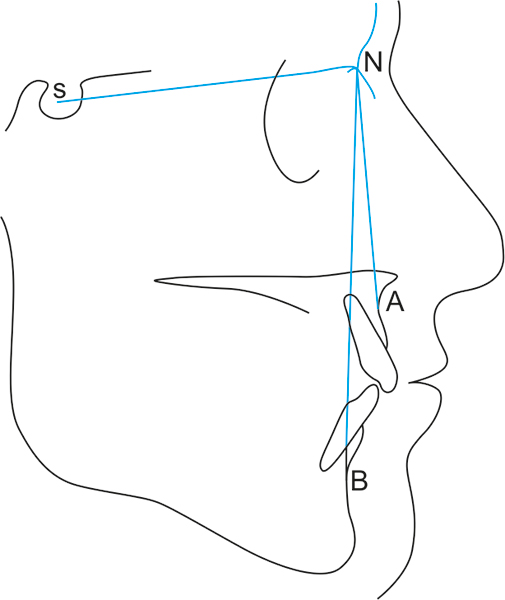The following landmarks were identified and traced:

• Point A: Subspinale

• Point B: Supramentale

• Point N: Nasion

• Point C: The apparent axis of the Condyle

• Point S: Midpoint of the sella turcica

• Point M: Midpoint of the premaxilla

• Point G: Center of the largest circle, i.e., at a tangent to the internal inferior, anterior, and posterior surfaces of the mandibular symphysis.

Lines and planes used in the analysis:

• N–A Line: Line connecting N and A points

• N–B Line: Line connecting N and B points

• Functional occlusal plane

• C–B Line: Line connecting the center of the condyle C with B point

• A–B Line: Line connecting A and B points

• Perpendicular line from point A: Line from point A perpendicular to the C–B line

• S–M Line: Line connecting S and M points

• M–G Line: Line connecting M and G points

• S–G Line: Line connecting S and G points

• Perpendicular line from point M: Line from point M perpendicular to the S–G line

The five parameters were measured as follows:

1. ANB angle (Fig. 1):

• • To construct ANB angle, points A, B, and N (Nasion) were located.

• • Two lines, N–A and N–B, were drawn.

• • ANB angle is between the N–A line and the N–B line at point N. Normal range of ANB angle is 0° to 4°. ANB > 4° is considered class II and ANB < 0° is skeletal class III relation.

2. Wits appraisal (Fig. 2):

AO–BO distance or Wits assessment is a linear distance between points A and B projected perpendicularly on the functional occlusal plane. In females, the normal value is 0 mm and in males it is –1 mm. In skeletal class II, point BO was positioned well behind point AO (positive reading), whereas in class III, point BO is ahead of point AO (negative reading).

##### Fig. 2:

Wits appraisal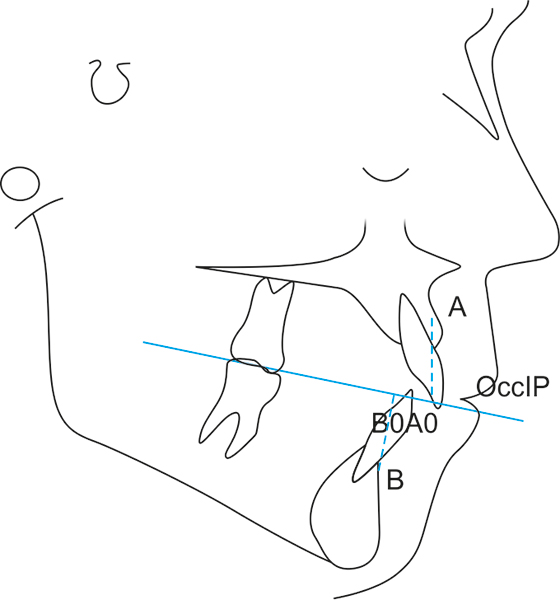##### Fig. 3:

Beta angle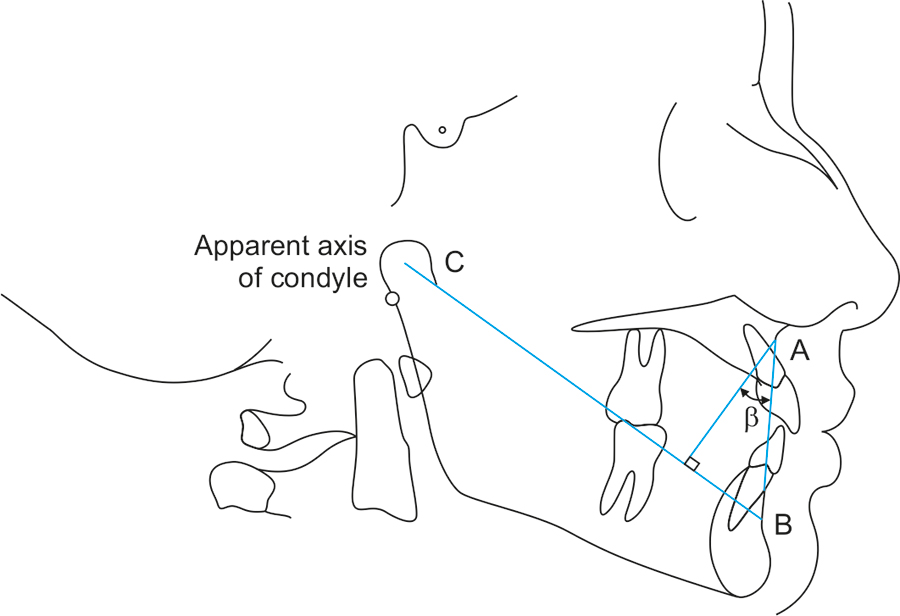3. Beta angle (Fig. 3):

• • To construct Beta angle, points A, B, and C (the apparent axis of condyle) were identified.

• • Three lines C–B, A–B, and a line from point A perpendicular to C–B line were drawn.

• • Beta angle is the angle between the perpendicular line and the A–B line. Beta angle between 27° and 35° can be considered as class I skeletal pattern. Angle <27° indicates class II and angle >35° indicates a class III skeletal pattern.

4. Yen angle (Fig. 4):

To construct Yen angle, points S, M, and G were located. G and M points were originally introduced by Nanda and Merrill16 and later used by Braun et al,17 being constructed at the center of the largest circle placed tangent to the anterior, superior (represented by nasal floor), and palatal surfaces of the premaxilla and the internal anterior, inferior and posterior surfaces at the mandibular symphysis. These points were not affected by local remodeling secondary to dental movements, unlike points A and B. A template with concentric circles whose diameters increased in 1 mm increments was used.

Two lines, S-M and M-G, were drawn. The Yen angle is measured between lines S–M and M–G at point M. Yen angle between 117 to 123° is considered as skeletal class I, Yen angle <117° is considered as class II, and Yen angle >123° is considered as skeletal class III.

5. W angle (Fig. 5):

To construct W angle, points S, M and G were identified.

Four lines, S–M, M–G, S–G, and a line from point M perpendicular to the S–G line, were drawn. The W angle is the angle between the perpendicular line from point M to S–G line and the M–G line. W angle between 51° and 56° is considered as class I skeletal pattern. W angle <51° is considered as skeletal class II relationship and W angle >56° is considered as skeletal class III relationship.

##### Fig. 4:

Yen angle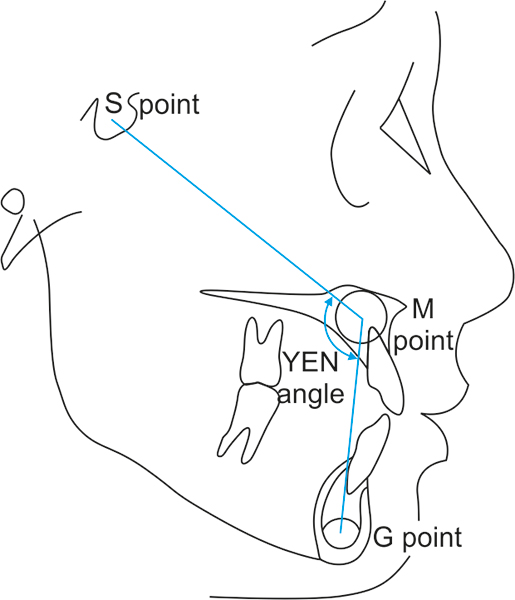##### Fig. 5:

W angle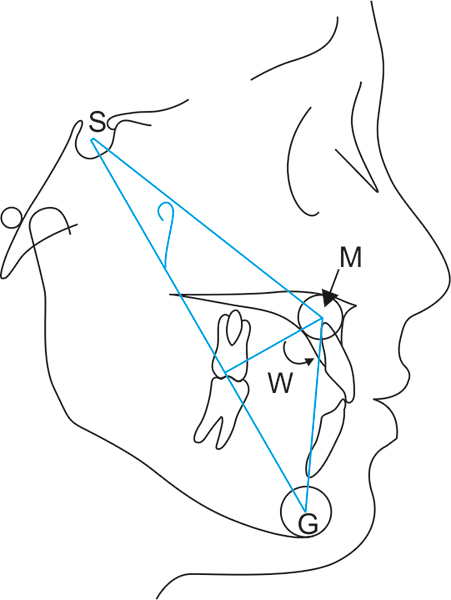After collection of the data, statistical analyses were calculated with independent t-test. Pearson's correlation coefficient, sensitivity, specificity, and accuracy were calculated using Statistical Package for the Social Sciences software version 13.0.

## RESULTS

Table 1 shows the descriptive statistics of age, ANB angle, Beta angle, Wits appraisal, Yen angle, and W angle for the sample of 40 subjects. The mean and standard deviation (SD) of Yen angle was found to be 120.20° ± 6.73°, whereas the mean and SD of W angle was found to be 52.97° ± 5.61°.

Table 2 shows the Pearson's correlation coefficient (r) among all the five sagittal jaw markers: ANB angle, Beta angle, Wits appraisal, Yen angle, and W angle in the overall sample. As can be seen, a significant correlation is found among all the five parameters. The r value of Yen angle is higher than that of W angle with ANB angle and Wits appraisal, whereas W angle shows higher value of correlation with Beta angle than that shown by Yen angle.

Table 1: Descriptive statistics of the variables

 Age (years) ANB angle (°) Beta angle (°) Wits appraisal (mm) Yen angle (°) W angle (°) Mean 20 4.27 28.15 2.82 120.20 52.97 Median 19.50 3.50 27.00 2.00 119.50 54.00 Std. deviation 1.90 3.30 6.95 4.05 6.73 5.61 Minimum 18.00 –5.00 5.00 –9.00 109.00 30.00 Maximum 26.00 10.00 47.00 14.00 140.00 64.00 Percentiles 25 (Q1) 19.00 2.00 24.25 0.62 114.50 51.00 50 (Q2) 19.50 3.50 27.00 2.00 119.50 54.00 75 (Q3) 21.00 7.00 33.00 4.00 124.75 56.00

Table 2: Correlation matrix for ANB angle, Beta angle, Wits appraisal, Yen angle, and W angle

 Beta angle Wits appraisal Yen angle W angle ANB angle –0.829* 0.778* –0.868* –0.756* Beta angle –0.550* 0.680* 0.755* Wits appraisal –0.620* –0.525* Yen angle 0.684*

*Correlation is significant at 0.01 level (two-tailed)

Table 3 shows the values of r2 for ANB angle, Beta angle, Wits appraisal, Yen angle, and W angle. As can be seen, Yen angle shows highest value of r2 with ANB angle (r= 0.753) and least with Wits appraisal (r= 0.384). Similarly, W angle also shows highest value of r2 with ANB angle (r= 0.571) and least with Wits appraisal (r= 0.275). It can also be noted that Yen angle shows better correlation with ANB angle and Wits appraisal, whereas W angle shows better correlation with Beta angle.

Thus, looking at the highest correlation of Yen angle and W angle with ANB angle, the sensitivity, specificity, and accuracy of Yen angle and W angle were calculated with ANB angle in the overall sample.

As seen in Table 4, both Yen angle and W angle show 100% sensitivity with ANB angle.

But, Yen angle shows better values of specificity and accuracy with ANB angle than that shown by W angle.

## DISCUSSION

Evaluation of sagittal jaw discrepancy is an indispensable step in every orthodontic diagnosis. Many angular and linear measurements have been devised till date, which uses different reference points and planes for this measurement. Different analyses may at times give different values for the same jaw relationship due to change in the reference planes. ANB angle, Wits appraisal, and Beta angle are the commonly used sagittal jaw indicators in regular orthodontic diagnosis. Yen angle and W angle have recently been introduced by Neela et al10 and Bhad et al11 respectively. In this study, an attempt was made to check these two new angles for their correlation with the routinely used measures, so as to assess their clinical use and predictability as sagittal jaw indicators.

Table 3: Values of r2 for all five sagittal jaw indicators

 Beta angle Wits appraisal Yen angle W angle ANB angle 0.687 0.605 0.753 0.571 Beta angle 0.302 0.462 0.570 Wits appraisal 0.384 0.275 Yen angle 0.467

Table 4: Sensitivity, specificity, and accuracy of W angle and Yen angle with ANB angle

 Sensitivity (%) Specificity (%) Accuracy (%) Yen angle 100 73.7 81.48 W angle 100 44.4 67.74

As can be seen in Table 1, the mean and SD values of Yen angle in this study were found to be 120.20° ± 6.73, which is similar to the values given by Neela et al,10 i.e., 119.79° ± 3.575 and that of W angle was found to be 52.97° ± 5.61, which is almost similar to that given by Bhad et al,11 i.e., 54.5° ± 4.09.

In Table 2, the correlation among all the five parameters is calculated, wherein it can be seen that they all show a significant correlation ( < 0.001) with each other, suggesting that all the five parameters can be successfully used in assessing the sagittal jaw discrepancy. This finding correlates with that of Mittal et al,12 Doshi et al,13 Trivedi et al,14 and Alam et al.15 ANB angle and Wits appraisal show a positive correlation with each other, but negative correlation with Beta angle, Yen angle, and W angle. This is due to the difference in the method of assessment used by these indicators. Further, it can be seen in Tables 3 and 4 and Graph 1 that Yen angle and W angle show a good correlation with each other with r = 0.684 and r= 0.467. This finding also correlates with that of Mittal et al12 (r = 0.735) and Trivedi et al14 (r = 0.894), who also found a good correlation between Yen angle and W angle.

##### Graph 1:

Scatter diagram showing correlation between Yen angle and W angle in overall sample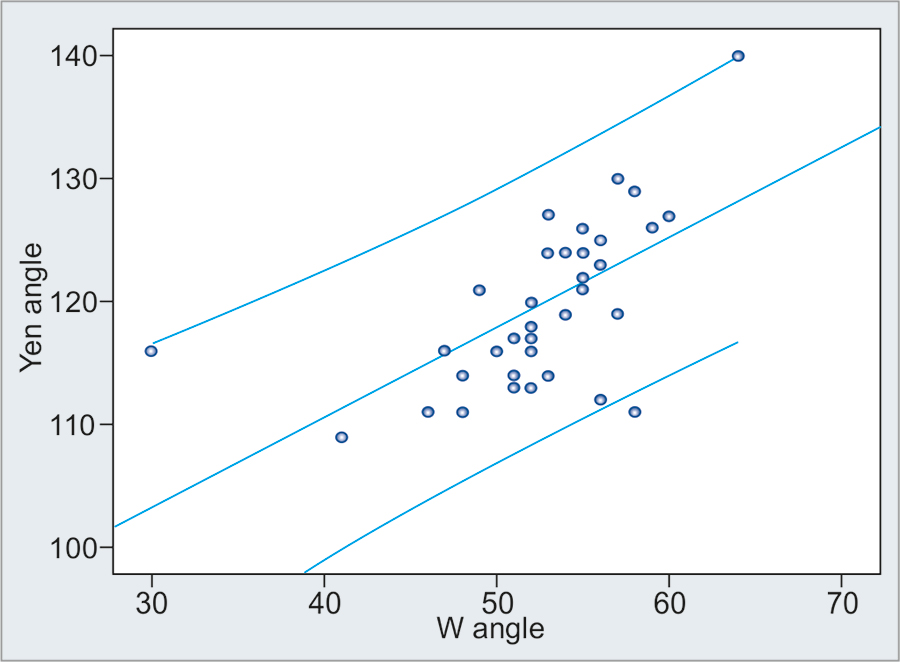##### Graph 3:

Scatter diagram showing correlation between W angle and ANB angle in overall sample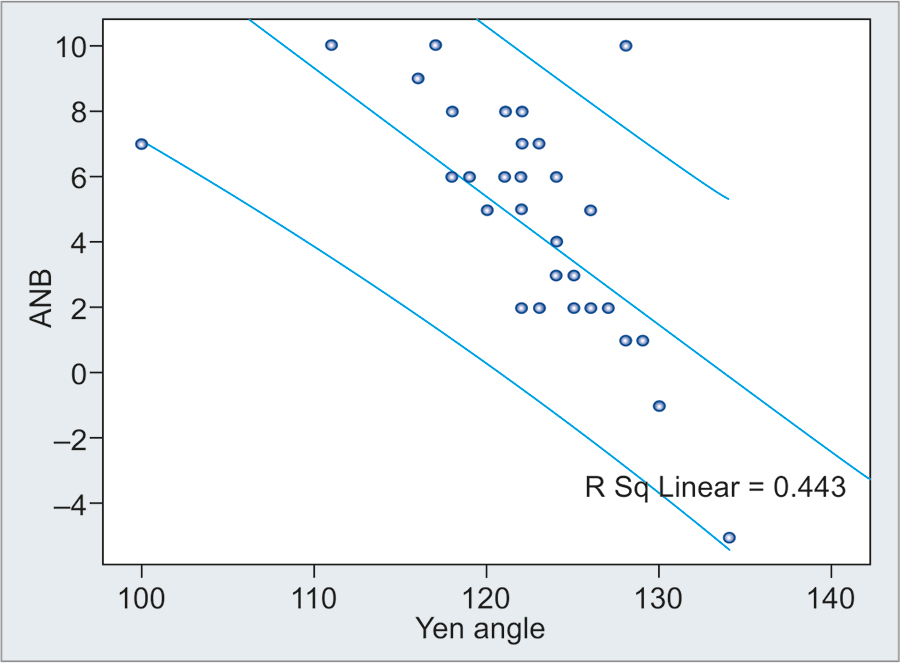Further, it can also be seen in Table 3 that Yen angle and W angle show best correlation with ANB angle with r= 0.753 and r= 0.571 respectively (Graphs 2 and 3), than either with Wits appraisal or Beta angle. The reason being that ANB angle, Yen angle, and W angle use a common reference “point S” for their measurements. The findings of Mittal et al12 also show a strong correlation of Yen and W angle with ANB angle, whereas the findings of Trivedi et al14 show a strong correlation only of W angle with ANB angle, but not of Yen angle.

It can also be seen further that Yen angle and W angle show least correlation with Wits appraisal with values of r= 0.384 and r= 0.275 respectively, although both ANB angle and Wits appraisal show a good correlation with each other with r= 0.605. This is in contrast to the findings of Mittal et al,12 where they found a strong correlation of Yen and W angle with Wits appraisal. A good correlation of Yen and W angle with Beta angle was also found in this study with r= 0.462 and r= 0.570 respectively. W angle shows better correlation with Beta angle than that shown by Yen angle. These findings coincide with that of Trivedi et al,14 who found a significant correlation between W angle with Beta angle, but not with Mittal et al,12 who did not find any significant correlation between these three parameters.

##### Graph 2:

Scatter diagram showing correlation between Yen angle and ANB angle in overall sample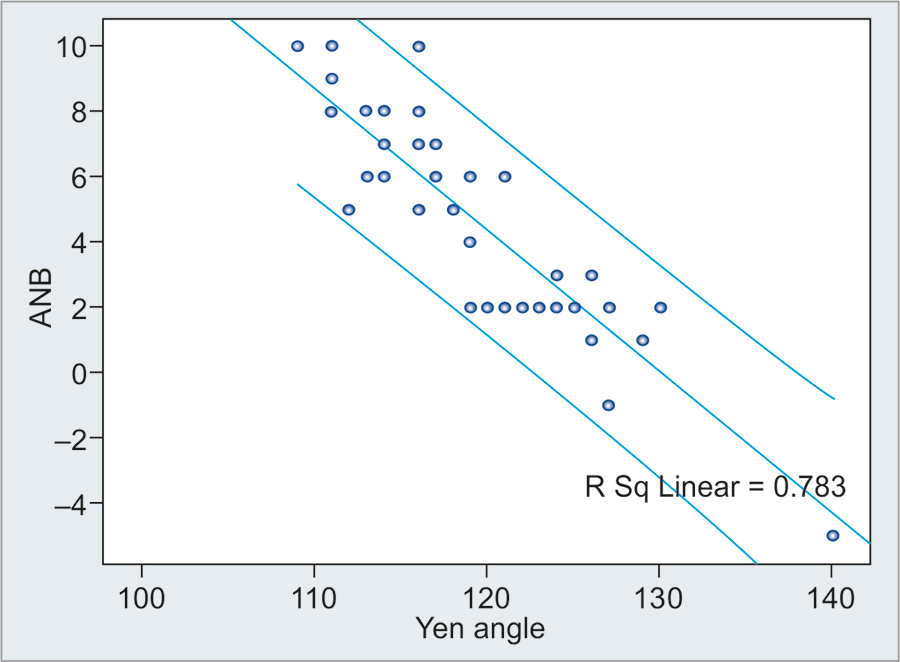Finally, sensitivity, specificity, and accuracy of W angle and Yen angle were calculated in relation to ANB angle (Table 4). In routine cephalometric analysis, ANB angle is the first choice for assessment of sagittal jaw discrepancy and is almost always calculated.16,17 It is a good sign that the assessment of Yen angle and W angle in this study showed a strong correlation with ANB angle. Both Yen angle and W angle showed 100% sensitivity with ANB angle in this sample. The values of specificity and accuracy of Yen angle with ANB angle are 73.7 and 81.48% respectively, whereas those of W angle with ANB angle are 44.4 and 67.74% respectively. Yen angle thus shows higher values of correlation, specificity, and accuracy with ANB angle than that shown by W angle. This finding also coincides with the study of Mittal et al,12 where Yen angle shows better correlation with ANB than that shown by W angle.

Thus, in this study, overall Yen angle shows better performance than W angle, which is in accordance with studies of Mittal et al,12 Doshi et al,13 and Trivedi et al,14 where Yen angle is shown to be a reliable and homogeneously distributed parameter to assess sagittal discrepancy.

Though ANB angle is the clinician's first choice in assessing the sagittal discrepancy of the jaws, it would be unjustified if the limitations of ANB are overlooked at this junction. The vertical height of the face, the anteroposterior position of nasion, and rotation of jaws either due to growth or treatment have a direct effect on the values of ANB. Do these factors show a similar impact on the values of Yen angle and W angle should be critically assessed using further studies.

Thus, it can be stated overall that as all the five sagittal indicators show a significant correlation with each other despite using different reference planes and methods of assessment, it would be justified to use more than one parameter for deciding sagittal dysplasia of any orthodontic case. Further, Yen angle and W angle can be used as sagittal jaw indicators in addition to the established measures, especially when alveolar remodeling during extensive anterior tooth movement is expected, as landmarks used in these angles are not influenced by that.

## CONCLUSION

• Despite varying strengths of association, statistically significant correlations were found among all the five measures; ANB angle, Wits appraisal, Beta angle, Yen angle, and W angle. Thus, Yen angle and W angle can be used to assess sagittal jaw discrepancy in addition to the established angles.

• Yen angle and W angle show highest correlation with ANB angle, whereas least correlation with Wits appraisal.

• Yen angle shows better values of specificity and accuracy with ANB angle than that shown by W angle.

##### Conflicts of interest

Source of support: Nil

Conflict of interest: None